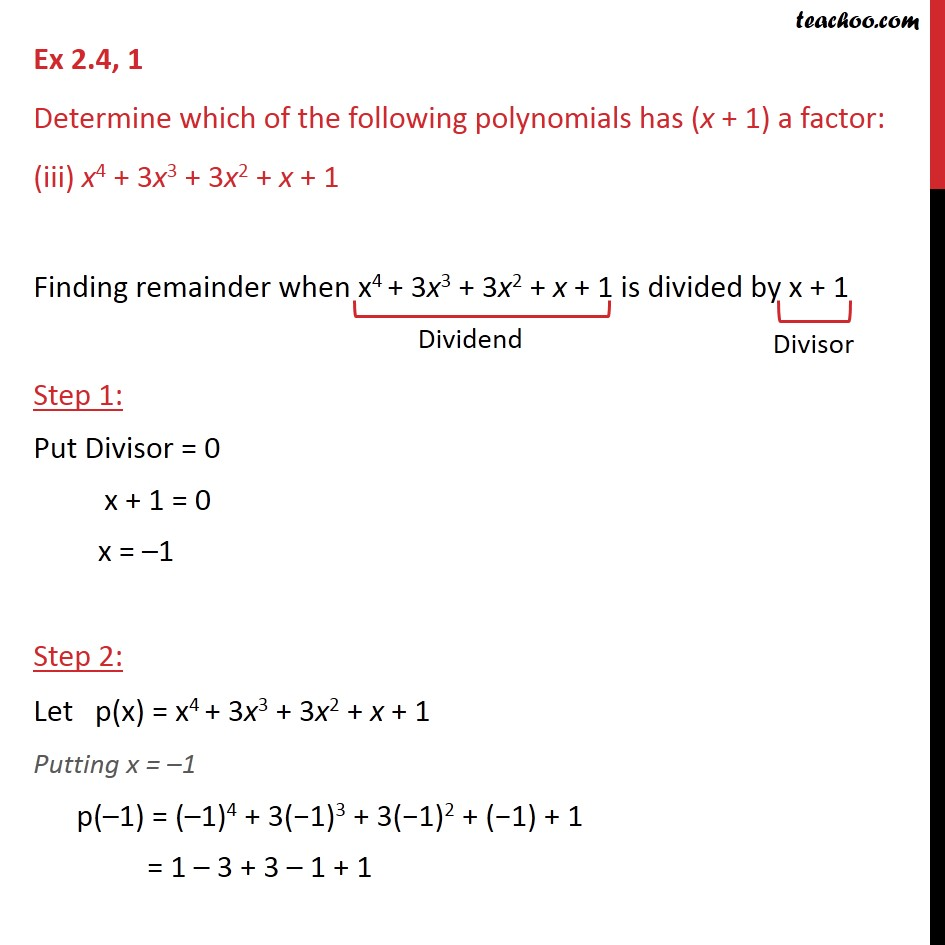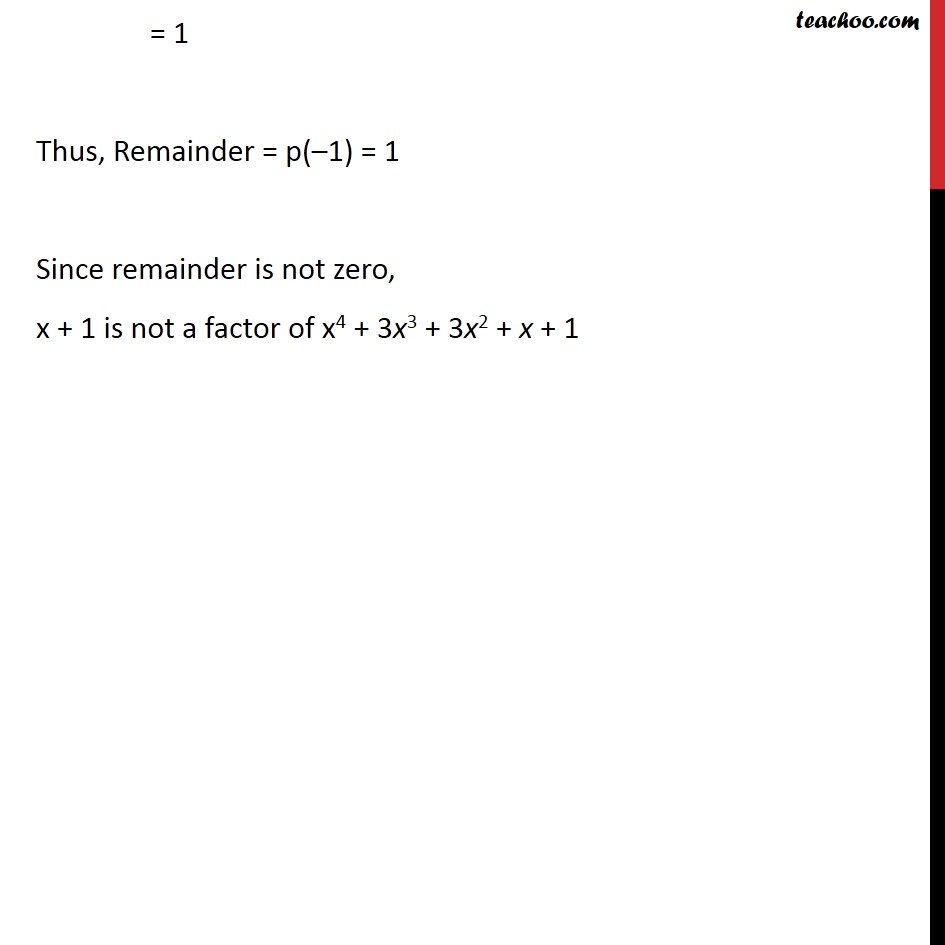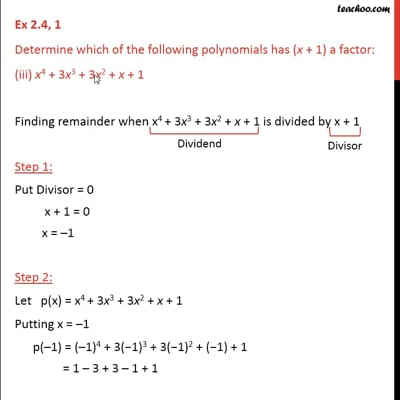Ex 2.4

Chapter 2 Class 9 Polynomials
Serial order wiseThis video is only available for Teachoo black users

Maths Crash Course - Live lectures + all videos + Real time Doubt solving!

### Transcript

Ex 2.4, 1 Determine which of the following polynomials has (x + 1) a factor: (iii) x4 + 3x3 + 3x2 + x + 1 Finding remainder when x4 + 3x3 + 3x2 + x + 1 is divided by x + 1 Step 1: Put Divisor = 0 x + 1 = 0 x = 1 Step 2: Let p(x) = x4 + 3x3 + 3x2 + x + 1 Putting x = 1 p( 1) = ( 1)4 + 3( 1)3 + 3( 1)2 + ( 1) + 1 = 1 3 + 3 1 + 1 = 1 Thus, Remainder = p( 1) = 1 Since remainder is not zero, x + 1 is not a factor of x4 + 3x3 + 3x2 + x + 1